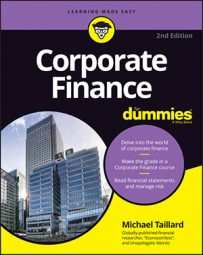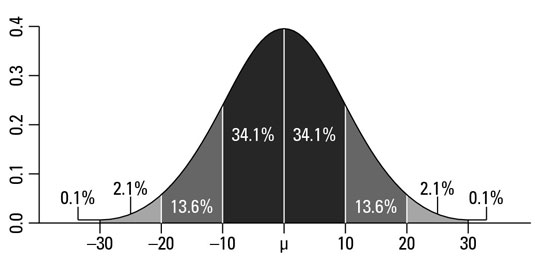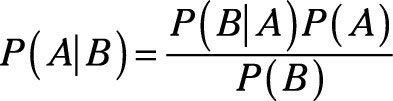##### Corporate Finance For DummiesProbability theory is pretty easy. The total probabilities of an event occurring or not will always equal 100 percent. If you have a 10 percent probability that something may happen, then you have a 90 percent probability that it won’t.

The simplest example is the coin toss. You have a 50 percent probability that the coin will land on either side because only two options exist. Take 100 percent probability, divide it by two options, and each option has only 50 percent probability. Each time you flip that coin, you have a 50 percent probability of it being heads or tails.

Just because it lands on heads 100 times in a row doesn’t mean the coin has a better chance of landing on tails: On flip 101, you still have a 50 percent probability that it will land on tails. (A lot of gamblers get stuck in that trap.)

When you apply probability theory to the standard deviation, you end up with something called a normal distribution.

The normal distribution has a lot of very important traits, but all you really need to know is the relationship between standard deviation, probability, and the distribution of data. The percentages in the curve itself tell you what percentages of the data are included within the number of standard deviation units listed at the bottom.

After you calculate the standard deviation and mean, you can figure out probability pretty easily. For example, say that you have a mean of 5 and a standard deviation of 1. According to the graph, 34 percent of all values will be between 5 and 6, 68 percent of all cases will be between 4 and 6, and so on.So, why on Earth do you care about normal distribution? You care because probability calculations are used frequently in financial forecasts. Say that you want to predict the most probable percentage drop in the stock market as a result of an increase in interest rates.

By collecting historical data and determining the mean and standard deviations, you can estimate the likely range to any percentage of probability you like. You might say that the stock market has a 68 percent probability of dropping by 1 to 2 percent or a 95 percent probability that it will drop between 0.8 to 2.2 percent.

The more certain you want to be, the wider your range is going to be because you have to account for a greater range of data that encompasses a particular level of probability.

To take this calculation a step further, say that you want to know the probability that, given the event that the stock market drops by 1 to 2 percent, what is the probability that a specific corporation’s stock will also drop by 1 to 2 percent? You can come up with the answer to this interesting question by using something called Bayesian Probability:This equation says that in order to calculate the probability of thing A happening conditionally of thing B, you take the following steps:

1. Take the probability of thing B happening as a result of thing A and multiply that amount by the probability of thing A.

2. Divide the answer by the probability of thing B happening.

In other words, if there is a 68 percent chance of the stock market decreasing by 1 to 2 percent (thing B) and only a 50 percent chance of a stock price drop happening without B (thing A), but a 95 percent probability that interest rates will rise given a stock price drop, then you can calculate the total probability of your stock dropping like this:

P = (.95*.50)/.68 = .698 or 70%

The probability that a drop in stock price given a drop in the market occurs is 70 percent.

After you get a chance to practice these conditional probabilities, they really are quite simple to perform and even modify to your own purposes. Conditional probabilities are used frequently in financial forecasting, often being incorporated in APT models and conditional performance projections.